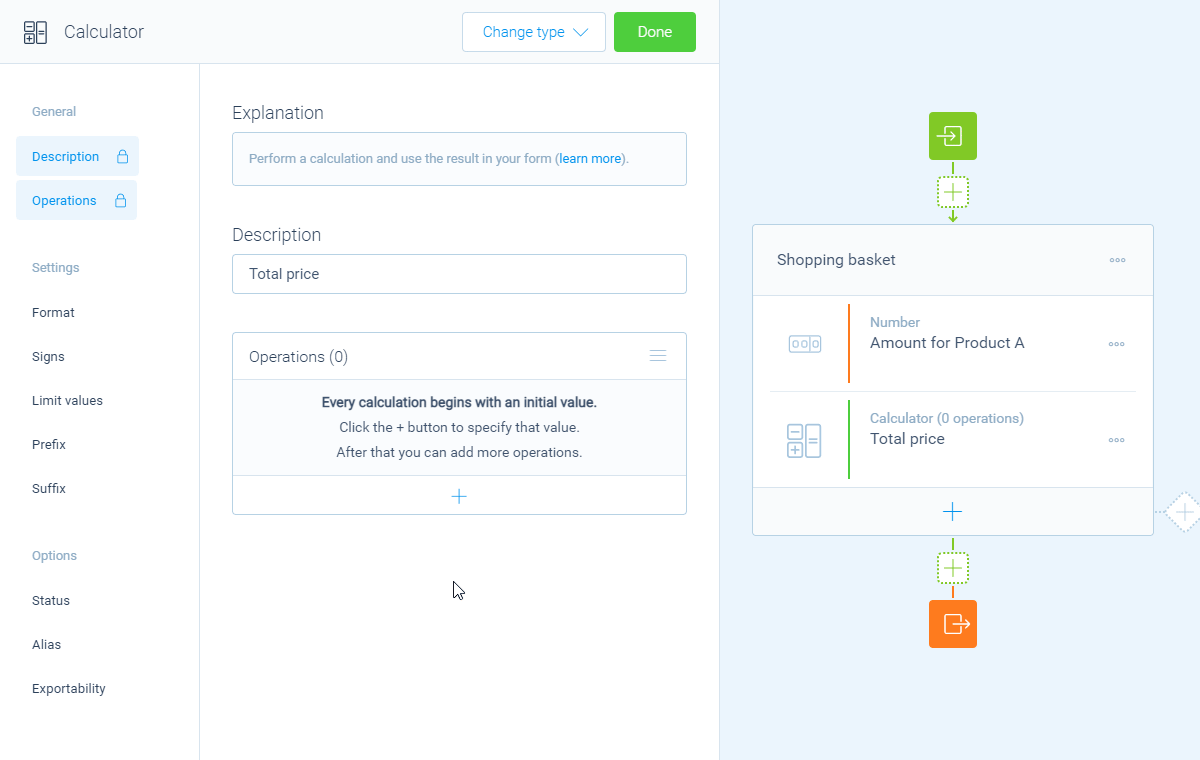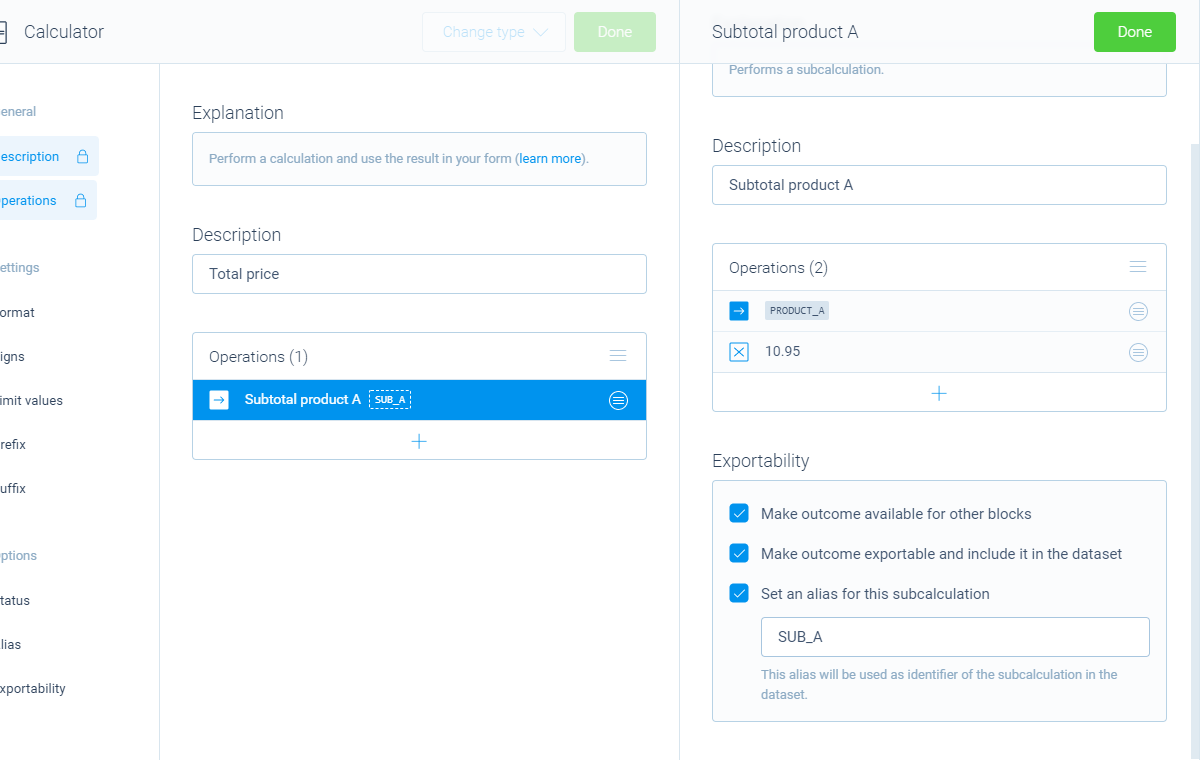Using logic4 Min.

# How to use subcalculations (multistep formulas) in your calculations

The calculator block is able to chain endless subcalculations to perform multistep formulas.

## When to use

Use the calculator block when you want to perform calculations inside your form. If you want to perform advanced calculations that require subcalculations, you can also do this. For example:

• First calculate the total price of selected products. And then use the result of that to do another operation;
• Perform a formula, for example to calculate the BMI: weight / (height*height).

These are just some examples. Basically you can calculate anything you want with the calculator block. Please have a look at our calculator features overview to see everything you can do with the calculator block.

## How to use

You can use a subcalculation inside each operation. From the menu to add an operation, you see an item called `Subcalculation` (if you have an empty form, you will see these menus directly). This adds a subcalculation to your calculator.

### Setup subcalculation

Each subcalculation includes ALL features that the calculator block offers, including all operations, scores, comparisons, functions and constants. Even including subcalculations, so you can use infinite and unlimited instances of subcalculations.In this example we use a subcalculation to calculate the subtotal of product A (\$10.95/piece).

### Exportability

You can determine if you want the result of the subcalculation to be available for other blocks and in your dataset, for example to show the outcome of the subcalculation to your respondent. The following settings are available:

• `Make answer available for other blocks` - Enable this to make the outcome of the subcalculation available to use in other blocks and even in logic branches;
• `Make answer exportable and include it in the dataset` - Enable this to save the outcome of the subcalculation to your dataset, so you can use it in results, exports, notifications and webhooks;
• `Set an alias for this answer` - Set an alias that gets used in the dataset (more information about aliases over here).You can determine if the outcomes of subcalculations are available and exportable.

The calculator block has lots of features, so we have several ways to learn all about it.

### Help center

Our help articles help you out on all different aspects of the calculator:

### Overviews

We also made some overviews of the capabilities that the calculator block provides: